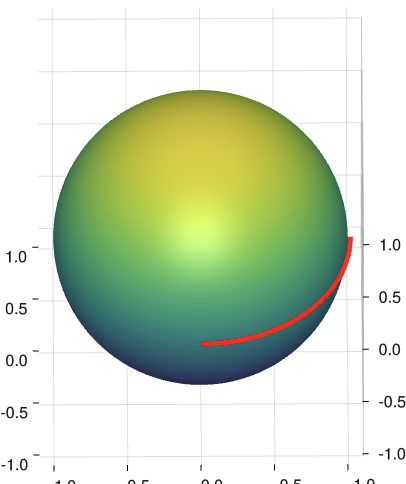# Makie.jl Fixed Perspective when Dragging Mesh

I’m using Makie.jl to generate a simple sphere. By default, the plot looks good but I would prefer if it did not resize as the user rotates the axis around. See the figure below for what I currently have.

``````f = Figure()
ax_sphere = Axis3(f[1,2], aspect=:data)
n = 50
θ = [0;(0.5:n-0.5)/n;1]
φ = [(0:2n-2)*2/(2n-1);2]
x = [cospi(φ)*sinpi(θ) for θ in θ, φ in φ]
y = [sinpi(φ)*sinpi(θ) for θ in θ, φ in φ]
z = [cospi(θ) for θ in θ, φ in φ]
surface!(ax_sphere, x, y, z)
``````The sphere on the right was rotated by the user and so the grid was resized. Ideally (after hiding the grid), the sphere stays a constant size no matter how it is rotated. I was looking for something similar to tau app which is exactly what I want.

That’s `ax.viewmode = :fit`, the default is `:fitzoom`

1 Like